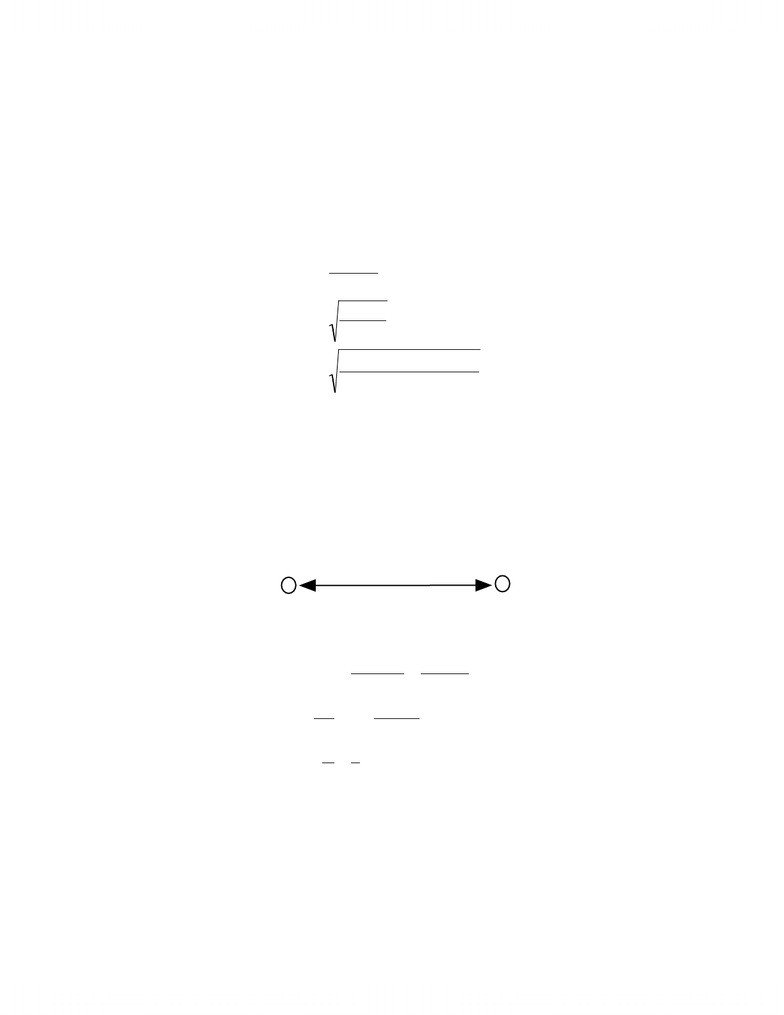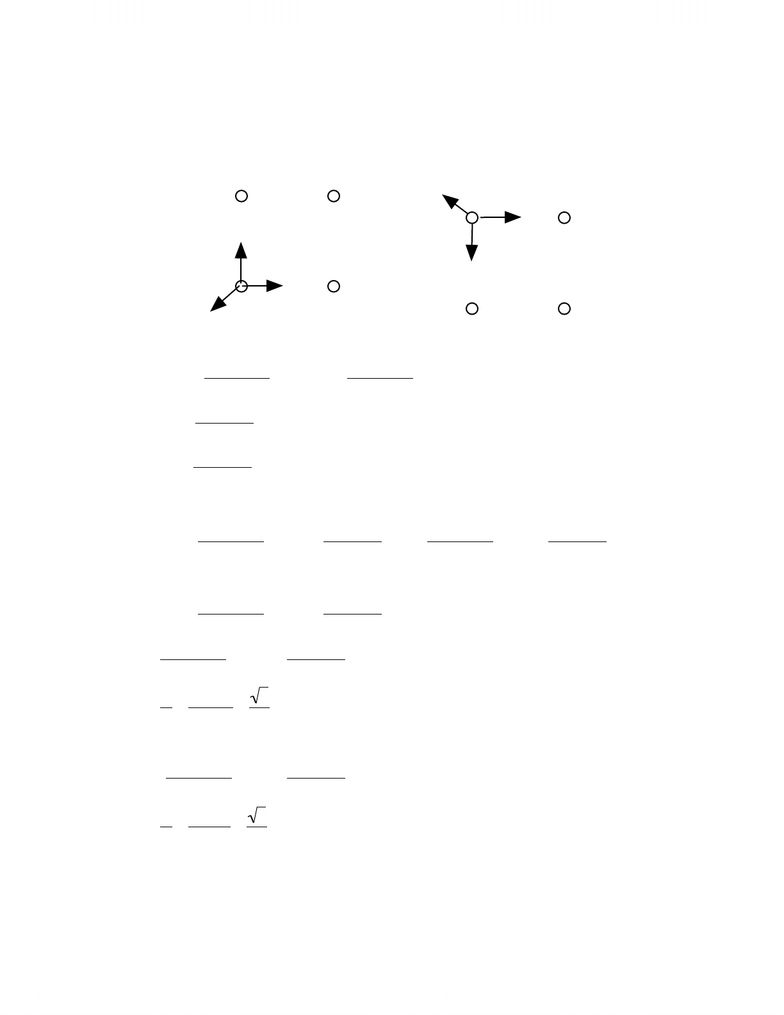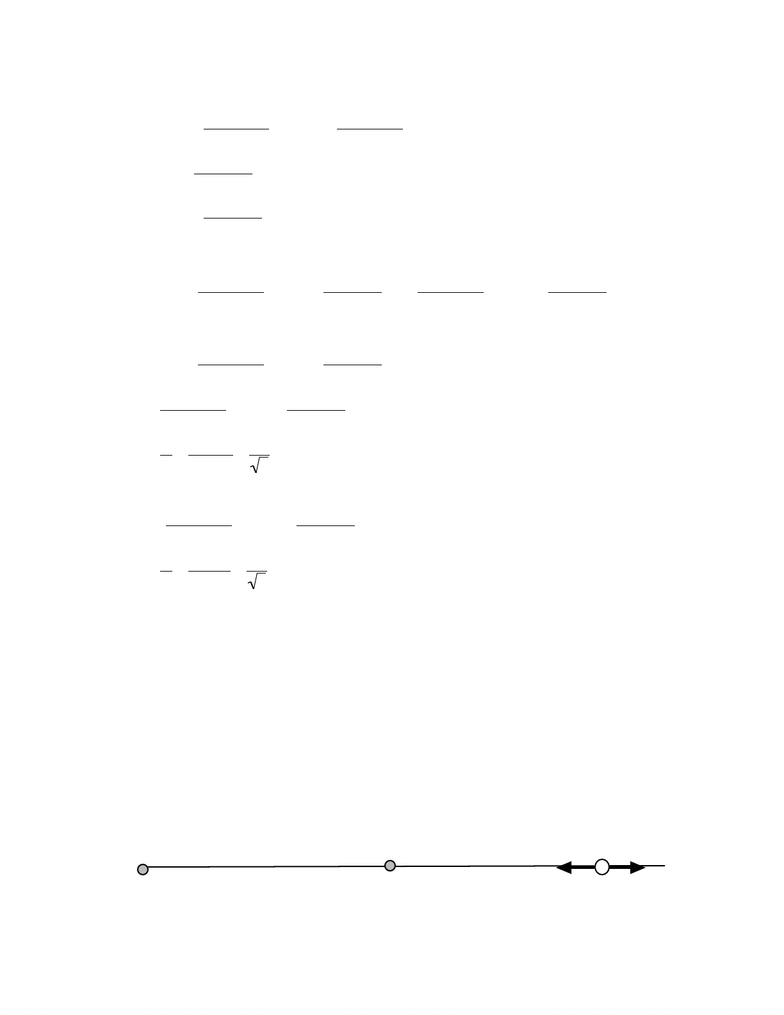Study Guides (390,000)
CA (150,000)
York (10,000)
PHYS (90)

# concept of phyics

Department
Physics and Astronomy
Course Code
PHYS 1010
Professor
Marko Horbatsch

This preview shows pages 1-3. to view the full 9 pages of the document.Chapter 21
21.1 What must the distance between point charge
q1=26 !10"6C
and point charge
q2=!47 "10!6C
for the electrostatic force between them to be 5.70N?
The magnitude of the force of attraction is given by Coulomb’s law where we have taken the
absolute value of the force and the charges.
F=q1q2
4
!"
0r2
r=q1q2
4
!"
0F
=26 #10\$6C%47 #10\$6C
4
!"
0%5.7N
=1.39m
21.5 Of the charge Q, on a tiny sphere, a portion q is to is to be transferred to a second nearby
sphere. The spheres can be treated as particles. For what value of q/Q maximizes the
magnitude F of the electrostatic force between the two spheres.
We begin with a drawing showing the charge on each sphere.
r
(Q-q) q
To ﬁnd the maximum, we take the derivative and set it equal to zero.
F=q(Q!q)
4
"#
0r2=Qq !q2
4
"#
0r2
dF
dq
=0=Q!2q
4
"#
0r2
q
Q
=1
2
21.8 In Fig 21-23, four particles for a square. The charges are
q1=q4=Q
and
q2=q3=q
(a) What is
Q/q
if the net electrostatic force on particles 1 and 3 is zero. (b) Is there any value
of q that makes the net electrostatic force on the each of the four particles zero? Explain.
www.notesolution.com

Only pages 1-3 are available for preview. Some parts have been intentionally blurred.We begin by drawing the forces on 1 and 3. We know that the diagonal force will be repulsive
and we know that the magnitude of the remaining forces are identical (since the charges are
identical). To get them to all balance, the q and Q must have opposite signs.
Qq
Q
q
F13
F23
F34
1 2
3 4
Q
q
Q
q
F31
F21
F41
1
2
3 4
!
F
23 =!q"q
4
#\$
0"2a2cos 45°ˆ
i +!q"q
4
#\$
0"2a2sin 45°ˆ
j
!
F
34 =q"Q
4
#\$
0"a2
ˆ
i
!
F
13 =q"Q
4
#\$
0"a2
ˆ
j
!
F =0=
!
F
23 +
!
F
34 +
!
F
13
0=!q"q
4
#\$
0"2a2cos 45°+q"Q
4
#\$
0"a2
%
&
'
(
)
* ˆ
i +!q"q
4
#\$
0"2a2sin 45°+q"Q
4
#\$
0"a2
%
&
'
(
)
* ˆ
j
x!component
0=!q"q
4
#\$
0"2a2cos 45°+q"Q
4
#\$
0"a2
%
&
'
(
)
*
q"q
4
#\$
0"2a2cos 45°=q"Q
4
#\$
0"a2
Q
q
=cos 45°
2
=2
4
y!component
q"q
4
#\$
0"2a2sin 45°=q"Q
4
#\$
0"a2
%
&
'
(
)
*
Q
q
=sin 45°
2
=2
4
We should now check the forces on charge 1.
www.notesolution.com

Only pages 1-3 are available for preview. Some parts have been intentionally blurred.!
F
41 =!Q"Q
4
#\$
0"2a2cos 45°ˆ
i +Q"Q
4
#\$
0"2a2sin 45°ˆ
j
!
F
21 =q"Q
4
#\$
0"a2
ˆ
i
!
F
31 =!q"Q
4
#\$
0"a2
ˆ
j
!
F =0=
!
F
41 +
!
F
21 +
!
F
31
0=!Q"Q
4
#\$
0"2a2cos 45°+q"Q
4
#\$
0"a2
%
&
'
(
)
* ˆ
i +Q"Q
4
#\$
0"2a2sin 45°+!q"Q
4
#\$
0"a2
%
&
'
(
)
* ˆ
j
x!component
0=!Q"Q
4
#\$
0"2a2cos 45°+q"Q
4
#\$
0"a2
%
&
'
(
)
*
Q"Q
4
#\$
0"2a2cos 45°=q"Q
4
#\$
0"a2
Q
q
=2
cos 45°
=4
2
y!component
Q"Q
4
#\$
0"2a2sin 45°+!q"Q
4
#\$
0"a2
%
&
'
(
)
*
Q
q
=2
sin 45°
=4
2
It does not appear possible to ﬁnd a ratio that will allow the force to be zero on 1 and 3 at the
same time. It is also not possible to make the net force on every particle zero at the same time.
21.11 In Fig. 21-24, three charged particles lie on the x axis. Particles 1 and 2 are ﬁxed in
place. Particle 3 is free to move, but the net electrostatic force on it from particles 1 and 2
happens to be zero. If
L
12 =L23
, what is the ratio
?
Again the forces must cancel. I will demonstrate another way to approach the problem
Allow the q’s to be either positive or negative. Let the charge at 3 be Q.
q1
q2
F1
F2
L12
L23
www.notesolution.com